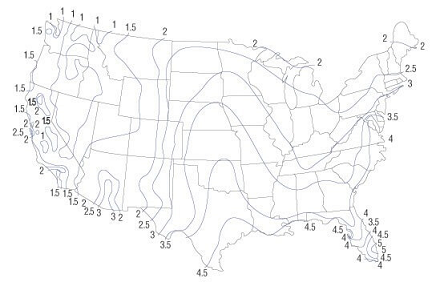# Rainwater Runoff (or) Storm Water Runoff Calculator Using Rational Method#Rainwater Runoff Calculator

This is to calculate and store your property rainwater runoff.

in
sq.ft# Rainwater Runoff Formulas

Q = (C x I x A )/96.23

Where,

Q is the storm-water Run-off in Gallons Per Minute (GPM)

C is the Run-off coefficient

I is the Rainfall intensity in inches per hour

A is the Drainage Area in square feet

96.23 is a conversion factor when you want the flow rate in GPM and your driange area in square feet

#### Hundred Year Rainfall MapThe above maps shows the rainfall in inches

###Recommended Pages ►

Richter to Tons of TNT Explosion

How far distance is that storm

Snow Weight Calculator

Volume of Rainfall Calculator

###More Calculators ►

Online math number calculation, formulas##Math Number Calculations ►

Online Algebra calculation, formulas , Digital calculation, Statistical calculation, Math Converters Pet Age Calculator,